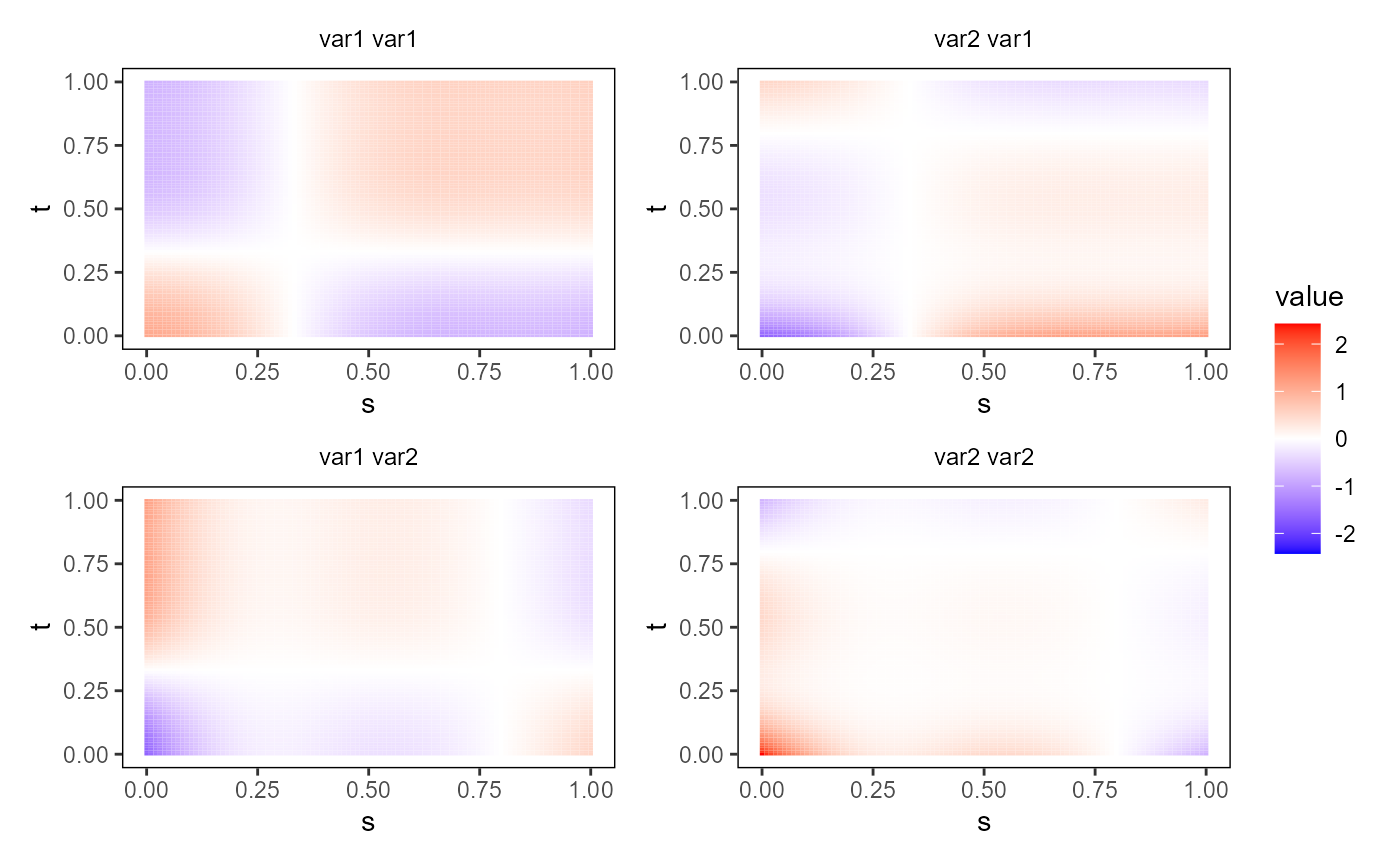Plot an object of class bifd using ggplot2 and geom_tile. The object must contain only one single functional replication.

plot_bifd(bifd_obj, type_plot = "raster", phi = 40, theta = 40)

## Arguments

bifd_obj

A bivariate functional data object of class bifd, containing one single replication.

type_plot

a character value If "raster", it plots the bivariate functional data object as a raster image. If "contour", it produces a contour plot. If "perspective", it produces a perspective plot. Default value is "raster".

phi

If type_plot=="perspective", it is the phi argument of the function plot3D::persp3D.

theta

If type_plot=="perspective", it is the theta argument of the function plot3D::persp3D.

## Value

A ggplot with a geom_tile layer providing a plot of the bivariate functional data object as a heat map.

## Examples

library(funcharts)
mfdobj <- data_sim_mfd(nobs = 1)
tp <- tensor_product_mfd(mfdobj)
plot_bifd(tp)